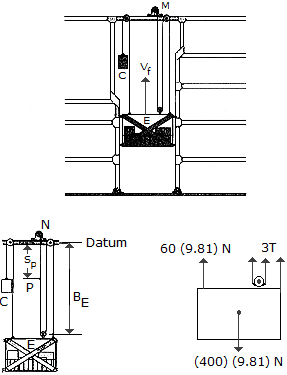# Engineering Mechanics - KOP: Work and Energy - Discussion

### Discussion :: KOP: Work and Energy - General Questions (Q.No.1)

1.The elevator E and its freight have a total mass of 400 kg. Hoisting is provided by the motor M and the 60-kg block C. If the motor has an efficiency of e = 0.6, determine the power that must be supplied to the motor when the elevator is hoisted upward at a constant speed of vE = m/s.

 [A]. P = 22.2 kW [B]. P = 13.34 kW [C]. P = 26.2 kW [D]. P = 30.1 kW

Explanation:

No answer description available for this question.

 Gokul Krsishnan said: (Nov 7, 2015) Please tell me the method used for solving this question.

 Chirag Sethi said: (Nov 24, 2015) Mc = 60. g = 9.8. Me = 400. E = 0.6. Ve = 4 must be to get an answer. F = (ME-MC)g. Power = FV/E. So Power = 22.2 KW.

 Binth Ismail said: (Oct 27, 2016) @Chirag. How do you get Ve = 4?

 Lakshmipathi said: (Aug 16, 2017) How you got Ve = 4?